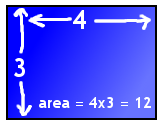# What is the area of a shape?

#### Quick Definition:

The area is the two-dimensional amount of space enclosed by a shape. For a square, it's literally the amount of "area" inside the edges of the square. For a three-dimensional object, we use the term surface area to represent the area of the outside surface of the shape.

#### Example:

The graphic below is a VERY simple example of the area of a rectangle. Multiplying the side lengths (3 times 4) gives us the area, 12. To think of it visually, 12 squares that are each 1x1 would fit in this area.